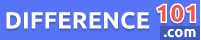# Mass vs. Weight: What Is The Difference Between Mass And Weight?

We often use the terms mass and weight interchangeably, but there is a difference between the two. Read on to learn more about the difference between these two measures, pros and cons of each.We all know what mass and weight are, but do we really know the difference between the two? Mass is the amount of matter in an object, while weight is a measure of the force of gravity on an object. The two are often confused, but they are actually quite different.

Mass is the measure of an object’s resistance to acceleration. It is also the measure of an object’s resistance to being deformed. This is why objects with more mass are harder to move and harder to change shape. Weight, on the other hand, is the measure of the force of gravity on an object. The force of gravity is what makes things fall to the ground.

So, mass is the amount of matter in an object, while weight is a measure of the force of gravity on an object.

## What Is Mass?

In physics, mass is a measure of the amount of matter in an object. It is also a measure of an object’s resistance to acceleration and the force required to change its velocity. The SI unit for mass is the kilogram.

👉  Minister vs. Pastor: What Is The Difference Between Minister And Pastor?

## What Is Weight?

Weight is a measure of the force of gravity on an object. The object’s weight depends on its mass and the strength of the gravitational field. The force of gravity is determined by the mass of the object and the distance between the object and the center of the Earth. The SI unit of weight is the newton.

## Key Differences Between Mass And Weight

There are a few key differences between mass and weight.

1. Mass is the amount of matter in an object, while weight is the force that gravity exerts on an object.
2. Mass is measured in kilograms (kg), while weight is measured in Newtons (N).
3. Mass is an inherent property of an object, while weight is a variable property that can change depending on the object’s location.
4. Mass is unaffected by gravity, while weight is affected by gravity.

## Mass vs. Weight Similarities

The two concepts are often confused, but they are actually quite different. Mass is a measure of the amount of matter in an object, while weight is a measure of the force exerted by gravity on an object. Despite this difference, there are some similarities between the two concepts. For one, both mass and weight are affected by the gravitational pull of the Earth; objects with more mass or more weight will be pulled more strongly by gravity. Finally, both mass and weight can be used to calculate the force of an object in motion; more massive or more heavily objects will have more force.

👉  Washington vs. Washington DC: Everything You Need To Know About The Difference Between Washington And Washington DC

## Mass Pros & Cons

### Mass Pros

1. Mass  is a very accurate measure.
2. It is relatively easy to determine the mass of an object, making it a convenient choice for many applications.
3. Mass is independent of the object’s environment.
4. Mass is a conserved quantity. This means that, under most circumstances, the mass of an object will remain constant. This is useful in many situations, as it allows for accurate measurements to be taken over long periods of time.

### Mass Cons

1. Mass is affected by the gravitational field in which an object is located, making it difficult to compare the mass of two objects in different locations.
2. Mass can be affected by other factors such as temperature; an object with a higher temperature will generally have a higher mass than an object with a lower temperature, even if they have the same amount of matter.
👉  What Is The Difference Between Kubernetes and Docker?

## Weight Pros & Cons

### Weight Pros

1. It is a very simple measurement to take.
2. Weight is a relatively constant force. It does not fluctuate as much as other forces, such as air resistance.
3. Weight can be used to calculate the density of an object. This is because the density of an object is equal to the mass of the object divided by the volume of the object.
4. Weight can be used to calculate the force of gravity between two objects. This is because the force of gravity is equal to the mass of the object times the acceleration due to gravity.

### Weight Cons

1. It is affected by the object’s environment. For example, an object on the Moon would weigh less than the same object on Earth because the force of gravity is weaker on the Moon.
2. Weight is affected by the object’s motion. An object at rest would weigh less than the same object in motion because of the effects of gravity.
3. Weight is a relative measure; two objects of different sizes and masses will have different weights, even if the force of gravity on them is the same.

## Conclusion

In conclusion, Mass can be defined as a measure of how much of an object/ substance/ substances there are. (e.g.: volume of a liquid). Weight on the other hand is a measure of the force of the object/substance.Michael Dorns

Michael Dorns is a media researcher and investigator at Difference 101. He graduated from California State University, Los Angeles, with a B.A. in English literature. He enjoys American literature, technology, animals, and sports. Michael has lived in four different countries on three continents and has also visited forty-two states and thirty-three countries. He currently resides in Los Angeles, California, with his wife and two children.

We will be happy to hear your thoughts